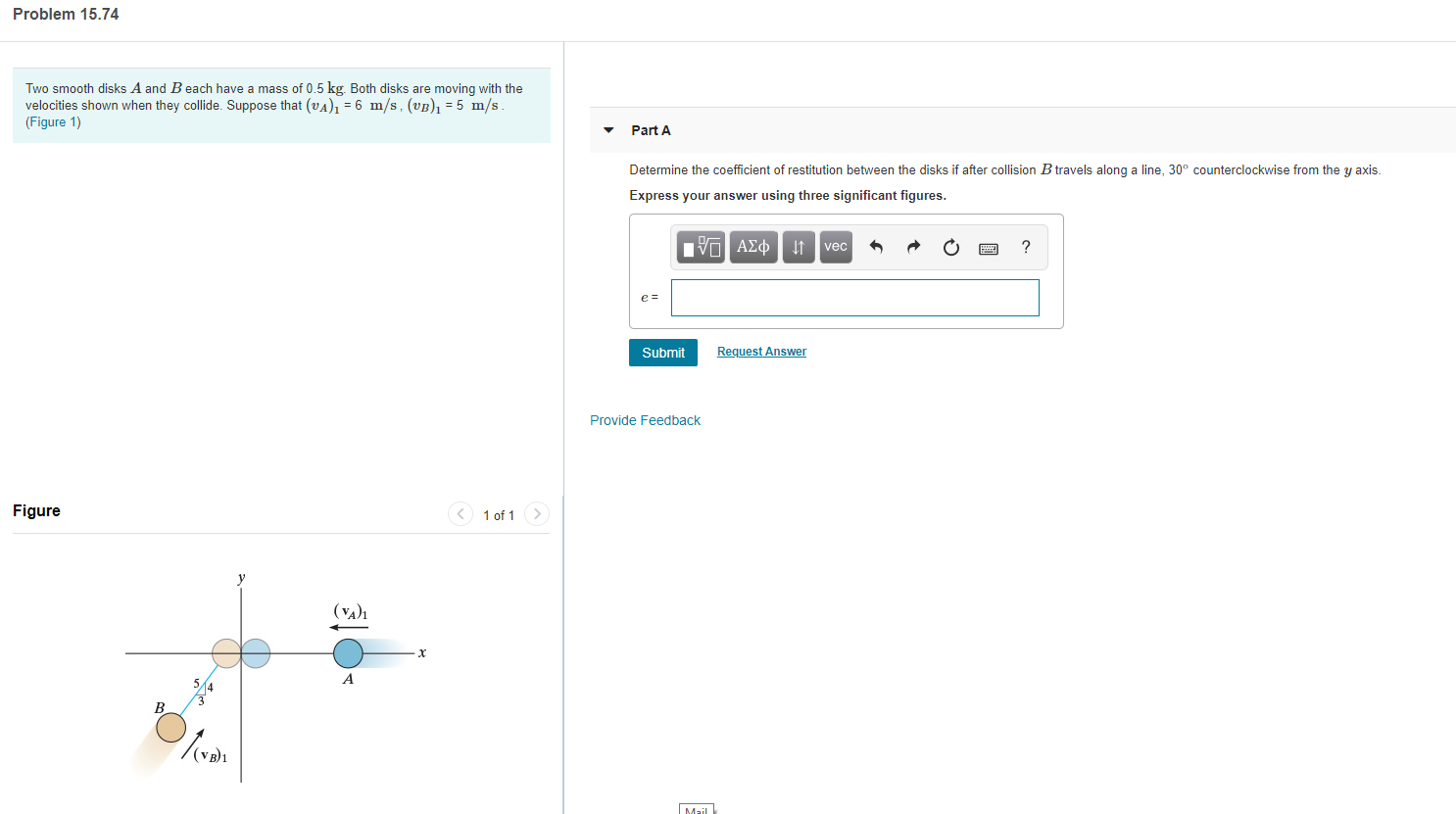# Problem 15.74 Two smooth disks A and B each have a mass of 0.5 kg. Both disks are moving with the velocities shown when they collide. Suppose that (vA) = 6 m/s, (vB) =5 m/s (Figure 1) Part A Determine the coefficient of restitution between the disks if after collision B travels along a line, 30° counterclockwise from the y axis Express your answer using three significant figures. nν ΑΣφ ? vec e = Submit Request Answer Provide Feedback Figure 1 of 1 (VA)1 X A B Mail

Questionhelp_outlineImage TranscriptioncloseProblem 15.74 Two smooth disks A and B each have a mass of 0.5 kg. Both disks are moving with the velocities shown when they collide. Suppose that (vA) = 6 m/s, (vB) =5 m/s (Figure 1) Part A Determine the coefficient of restitution between the disks if after collision B travels along a line, 30° counterclockwise from the y axis Express your answer using three significant figures. nν ΑΣφ ? vec e = Submit Request Answer Provide Feedback Figure 1 of 1 (VA)1 X A B Mail fullscreen

1 Rating

### Want to see this answer and more?

Experts are waiting 24/7 to provide step-by-step solutions in as fast as 30 minutes!*

*Response times vary by subject and question complexity. Median response time is 34 minutes and may be longer for new subjects.
Tagged in
EngineeringMechanical Engineering

### Classical Mechanics